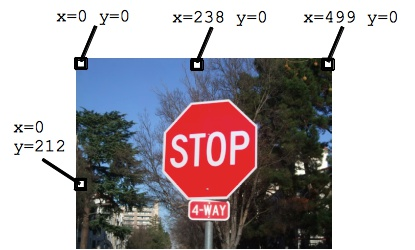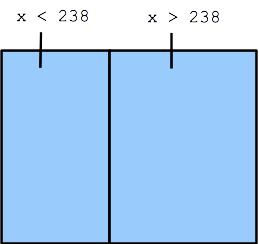# Image-7 If Logic

• Theme 1: Loop bulk operation - e.g. for-loop
- Loop body code runs again and again
• Theme 2: Logic test true/false - e.g. if-statement (this section)
- Run some code only if a test is true

Our use of loops thus far have allowed us to write a little bit of code which is run for many data points. That's one big theme in computer code. The if-statement, the topic of this section, will add a second theme: the ability to write a true/false test to control if a bit of code runs or not. Combined with the loop, the if-statement will greatly expand what we can do with code.

## Image X/Y Refresher• Today we'll use the stop sign image
• 500 pixels wide, 375 pixel high
• Every pixel is identified by an x,y coordinate
• x=0 y=0 is the upper left pixel, aka (0, 0)
• X values increase going to the right
• Y values increase going down

## pixel.getX() pixel.getY() image.getWidth() image.getHeight()

• Pixels x/y functions: `pixel.getX()` and `pixel.getY()`
• These retrieve the X and Y values of the pixel
• Analogous to `pixel.getRed()`
• There are also `image.getWidth()` and `image.getHeight()`
• e.g. image.getWidth() on the stop sign image yields 500
• For now, you may type in literal numbers like 500

## If-Statement Demo

The if-statement has a true/false test which controls if some code is run or not. Here is an example if-statement shown inside a for-loop with the "stop.jpg" image.

image-logic-1

• 1. `if (pixel.getX() < 100) {` - "test" inside parenthesis
• 2. Curly braces around body code after the test `{ .. }` (indented)
• How works: the loop hits every x,y. Only some x,y pass the test.
• For now just using < (less than) or > (greater than) in test
• Experiment 1: try pixel.getX() < 150 300 10
• Experiment 2: now try pixel.getY() < 100 200
• The Flip
• Change < to >, .e.g. `(pixel.getX() > 100)`
• Get the opposite (well, very close to the opposite)

## Flip Diagram## If-Statement - You Try It

• Figure out code for the following
• stop.jpg is 500 pixels wide, 375 pixel high
• a. Set approximately the left 2/3's of the image to pure blue, just enough so none of the stop sign is visible
• b. Set a vertical stripe 20 pixels wide at the far right to blue
• c. Set a horizontal stripe 20 pixels high across the top of the image to black
• d. Set the flip of (c) to black, i.e. all except the top horizontal stripe to black

image-logic-2

Solution code

## Using image.getWidth() Expressions (optional)

• The "stop.jpg" image is 500 pixels wide
• Therefore to paint the left half, the test uses 250, as below
• The code uses 250 since that is 500/2
- instead of 250 type in below `image.getWidth()/2`